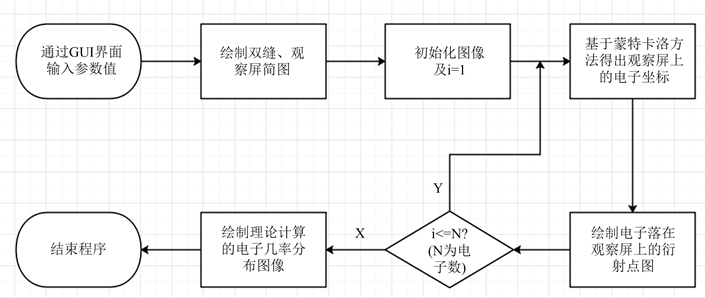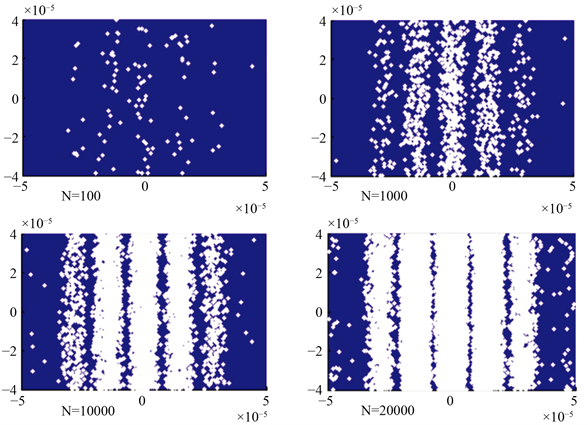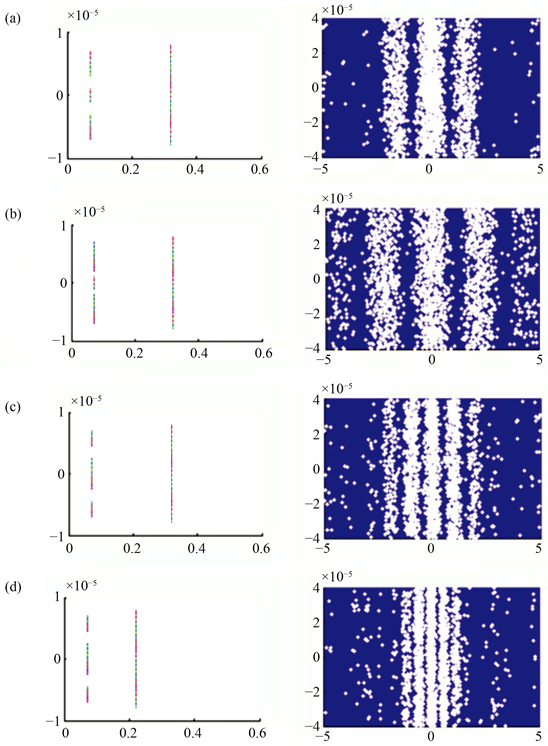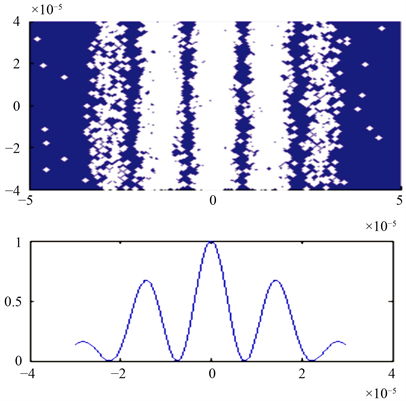﻿ Matlab在《原子物理学》教学中的应用——以电子双缝衍射实验为例

# Matlab在《原子物理学》教学中的应用——以电子双缝衍射实验为例Application of Matlab in Atomic Physics Teaching—Taking Electron Double Slit Diffraction Experiment as an Example

Abstract: The atomic physics course is a science based on experiments. We do numerical simulation of re-lated experiments with the help of Matlab to demonstrate experimental phenomena and experi-mental results. These can help students understand physical images and concepts, cultivate stu-dents’ innovative thinking and ability in science and technology, improve the quality of classroom teaching and stimulate students’ interest in learning.

1. 引言

2. 电子双缝衍射概率模型的构建

2.1. 德布罗意假设概率模型   

$\lambda =\frac{h}{p}=\frac{h}{mv}$ (1)

$v\ll c$ (c为光速)时，电子波的波长为：

$\lambda =\frac{h}{\sqrt{2meU}}$ (2)

$I\left(x\right)={I}_{0}\frac{{\mathrm{sin}}^{2}\left(\frac{\pi a}{\lambda }\mathrm{sin}\theta \right)}{{\left(\frac{\pi a}{\lambda }\mathrm{sin}\theta \right)}^{2}}{\mathrm{cos}}^{2}\left[\frac{\pi \left(a+b\right)}{\lambda }\mathrm{sin}\theta \right]$ (3)

$\mathrm{sin}\theta \approx \mathrm{tan}\theta =\frac{x}{D}$ (4)

$I\left(x\right)={I}_{0}\frac{{\mathrm{sin}}^{2}\left(\frac{\pi a}{\lambda D}x\right)}{{\left(\frac{\pi a}{\lambda D}x\right)}^{2}}{\mathrm{cos}}^{2}\left[\frac{\pi \left(a+b\right)}{\lambda D}x\right]$ (5)

$K=\frac{I\left(x\right)}{{I}_{0}}=\frac{{\mathrm{sin}}^{2}\left(\frac{\pi a}{\lambda D}x\right)}{{\left(\frac{\pi a}{\lambda D}x\right)}^{2}}{\mathrm{cos}}^{2}\left[\frac{\pi \left(a+b\right)}{\lambda D}x\right]$ (6)Figure 1. Experimental apparatus for electron double slit diffraction

2.2. 量子力学概率模型   

$\Psi \left(x,t\right)={\Psi }_{0}{\text{e}}^{\frac{\text{i}}{\hslash }\left(pz-Et\right)}$ (7)

$\Psi \left(x,t\right)=2{\Psi }_{0}\frac{\mathrm{sin}\left(k\frac{a}{2}\mathrm{sin}\theta \right)}{k\frac{a}{2}\mathrm{sin}\theta }\mathrm{cos}\left[k\frac{a+b}{2}\mathrm{sin}\theta \right]{\text{e}}^{\frac{\text{i}}{\hslash }\left(pz-Et\right)}$ (8)

$\omega \left(x\right)={|\Psi \left(x,t\right)|}^{2}=4{|{\Psi }_{0}|}^{2}\frac{{\mathrm{sin}}^{2}\left(\frac{\pi a}{2}\mathrm{sin}\theta \right)}{{\left(\frac{\pi a}{2}\mathrm{sin}\theta \right)}^{2}}{\mathrm{cos}}^{2}\left[k\frac{\left(a+b\right)}{2}\mathrm{sin}\theta \right]$ (9)

${\omega }_{\text{0}}\text{=}{|{\Psi }_{\text{0}}|}^{\text{2}}$，则

$\omega \left(x\right)={\omega }_{0}\frac{{\mathrm{sin}}^{2}\left(\frac{\pi a}{\lambda }\mathrm{sin}\theta \right)}{{\left(\frac{\pi a}{\lambda }\mathrm{sin}\theta \right)}^{2}}{\mathrm{cos}}^{2}\left[\pi \frac{\left(a+b\right)}{\lambda }\mathrm{sin}\theta \right]$ (10)

$D\gg \left(a+b\right)$，则有

$\mathrm{sin}\theta =\frac{x}{D}$ (11)

$\omega \left(x\right)={\omega }_{0}\frac{{\mathrm{sin}}^{2}\left(\frac{\pi a}{\lambda D}x\right)}{{\left(\frac{\pi a}{\lambda D}x\right)}^{2}}{\mathrm{cos}}^{2}\left[\frac{\pi \left(a+b\right)}{\lambda D}x\right]={\omega }_{0}\frac{{\mathrm{sin}}^{2}\left(Ax\right)}{{\left(Ax\right)}^{2}}{\mathrm{cos}}^{2}\left(Bx\right)$ (12)

3. Matlab计算结果与分析

3.1. 电子双缝衍射实验程序设计

3.2. 不同电子数目的衍射图案对比Figure 2. Matlab simulation program flow chart of electron double slit diffractionFigure 3. Diffraction pattern for N = 100, 1000, 5000, 20,000

3.3. 缝宽及双缝间距对衍射图案的影响Figure 4. (a) Diffraction pattern with crack width of 0.2 μm, double slit spacing of 0.2 μm and D of 0.25 m; (b) Diffraction pattern with crack width of 0.1 μm, double slit spacing of 0.2 μm and D of 0.25 m; (c) Diffraction pattern with crack width of 0.1 μm, double slit spacing of 0.5 μm and D of 0.25 m; (d) Diffraction pattern with crack width of 0.1 μm, double slit spacing of 0.2 μm and D of 0.15 m

3.4. 衍射图像与几率分布函数图像的对比Figure 5. The diffraction probability density image corresponding to the electron double slit diffraction experimental image

3.5. 电子衍射图像与波函数

4. 教学效果测评Table 1. Comparison of final grades of atomic physics courses

5. 结论

 高娟, 孙亚娟, 圣宗强, 李洋, 王兵. 基于翻转课堂的PBL教学法在原子物理课程教学中的研究[J]. 创新教育研究, 2020, 8(5): 708-712.

 陈昌兆, 王兵, 张晓森. 拼图式教学法在原子物理学课堂教学中的应用研究[J]. 大学物理, 2015, 34(11): 47-56.

 杨福家. 原子物理学[M]. 第4版. 北京: 高等教育出版社, 2008.

 储圣麟. 原子物理学[M]. 北京: 高等教育出版社, 2019.

 周佩瑶, 武星. 单电子双缝及多缝衍射的定量分析[J]. 天津大学学报, 2002, 35(2): 250-252.

Top